Equation Editor
•  941,475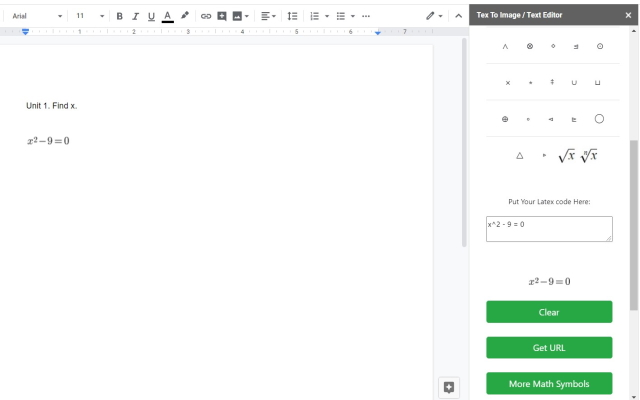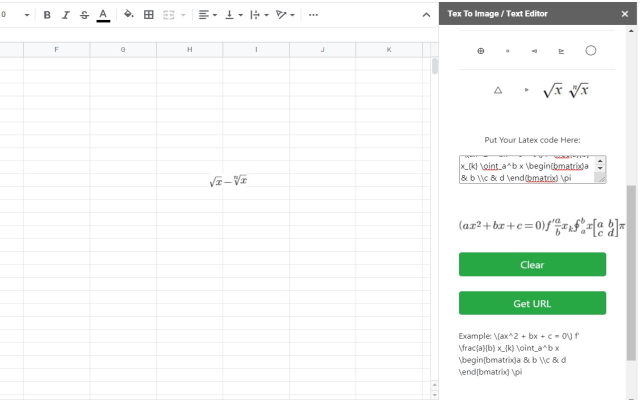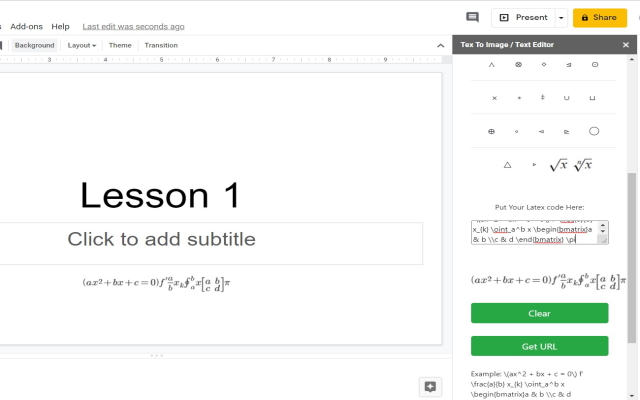Overview
Edit and add in math formulas inside Google Documents/Google Slides. Write math formulas for homework, exams and teaching materials.
```Our app is a free online add on to add and edit math formulas. You need to prepare for for your studies, exams and teaching materials.

Ever wanted a simple method to write math equations without having to use another app/software? Our addon will help you to write math equations easily on the computer.

You can add and edit math formulas very simply and intuitively, then save the output file as png file format and insert this math equation image to your Google Docs/Google Slides.

To begin using the software, simply do the following. Click on Addons - Equation Editor for Maths - and our addon sidebar will open up. Choose the math symbols you need to use to begin writing out your math equation. Once you have finished writing out your math equation, press the save button and our software will save this math equation as an image.
Common Math Symbols which are provided include:
Greater then or equal to
Square Root
To the power of
Fractions
and much more...

This is a lite, free & flexible LaTeX editor app for your Google Documents, Slide, Sheet.```
Reviews
Language:
Sort by:A User of Equation Editor for Maths
October 18, 2020
This is a great tool
Yes
NoA User of Equation Editor for Maths
October 9, 2020
Not even opening..disappointed
Yes
No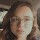A User of Equation Editor for Maths
October 3, 2020
I installed this app and when I hovered over it in my google docs, it just said "help" witch took me to how to install it, which was already done. This application does not work. I wouldn't suggest this to anyone else who is looking for a math add on. I have been able to open one that says "mathtype" though. it should be located at the very top.
Yes
No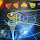A User of Equation Editor for Maths
October 1, 2020
I can't open it.
Yes
No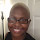A User of Equation Editor for Maths
September 24, 2020
i cant even open it! >:(
Yes
No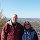A User of Equation Editor for Maths
September 8, 2020
Can't open it. I was looking for Math Type in Add-ons which took me to a mailmerge add-on. I thought this might have been a substitute.
Yes
No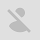A user of Equation Editor for Maths
September 23, 2020
this is the bomb IDEA.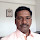A User of Equation Editor for Maths
September 5, 2020
I am a new user
Yes
NoA user of Equation Editor for Maths
September 9, 2020
will it answer the math problems?A User of Equation Editor for Maths
August 30, 2020
fake app. nothing
Yes
No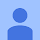A User of Equation Editor for Maths
August 12, 2020
Yes
NoA user of Equation Editor for Maths
August 18, 2020
does not workA User of Equation Editor for Maths
August 8, 2020
i'd give it a zero if i could. don't waste your time.
Yes
NoA user of Equation Editor for Maths
September 7, 2020
hey
1-10 of 17